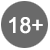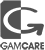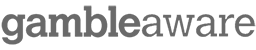# BankrollMob Forum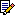BankrollMob Forum » Poker Forum » Estimating true win rate for ring games

 Estimating true win rate for ring games +1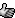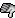just a basic way to estimate your true win rate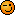if
sample size = n hands
average win rate(mean) = x bb/hand
standard deviation = s bb/hand

then
standard error of mean = e = s / (n^0.5)

now for 95% confidence limits-
true win rate = x +/- 2e

******************************************************
95% confidence limits means - your true win rate will lie within the given range approx 95% of time

******************************************************
most of the tracking software provide data /100 hands so
if
sample size = n hands
effective sample size = N = n/100
average win rate (mean)= X bb/100 hands
standard deviation = S bb/100 hands

then
standard error of mean = E = S/(N^0.5)

now for 95% confidence limits-
true win rate = X +/- 2E

******************************************************
converting /100 hand values to /hand

win rate per 100 hands (X) = 100 * win rate per hand (x)
standard deviation per 100 hands (S) = 10 * standard deviation per hand (s)

note- to analyze a data, both mean and standard deviation should have same units.

******************************************************

limitations and assumptions -

- standard deviation was calculated by stdevpa/stdeva function in microsoft excel (or equivalent)
- sample is representative, meaning you assume your opponents, their skill level, your skill level, your playing style etc will remain same in future.
so that standard deviation of sample is equivalent to standard deviation of population.
- sample size << population size
sample size should not be exceeding 5% of population size for which these results are assumed to be applicable.
- sample size should be large enough so that expected sampling distribution of means of sample size n or N follow a normal (or near normal) distribution.
how much size is sufficient is kinda debatable.
for any kind of distribution without outliers, 40-50 is considered minimum.
but outliers are pretty common in poker, so you need a bigger size.
i think for /hand, n >500 and for /100 hand analysis N>100

******************************************************
questions welcome0Poker = math = fact, you just proved it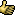BankrollMob Forum » Poker Forum » Estimating true win rate for ring games

Forum Rules | Support & FAQ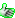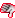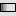Please Play Responsibly